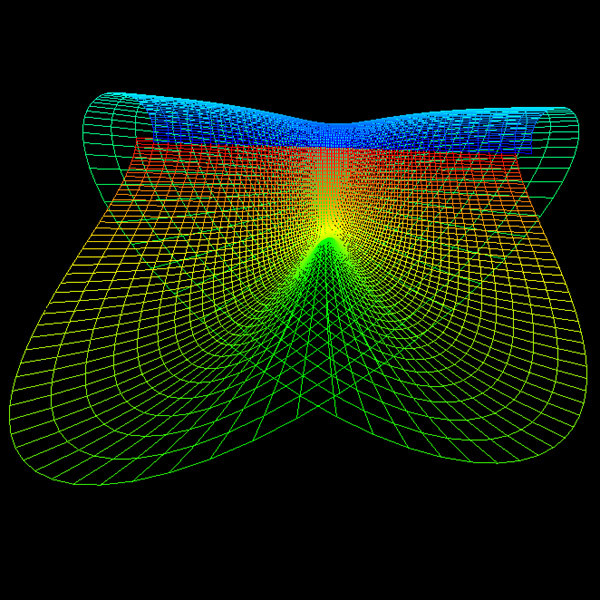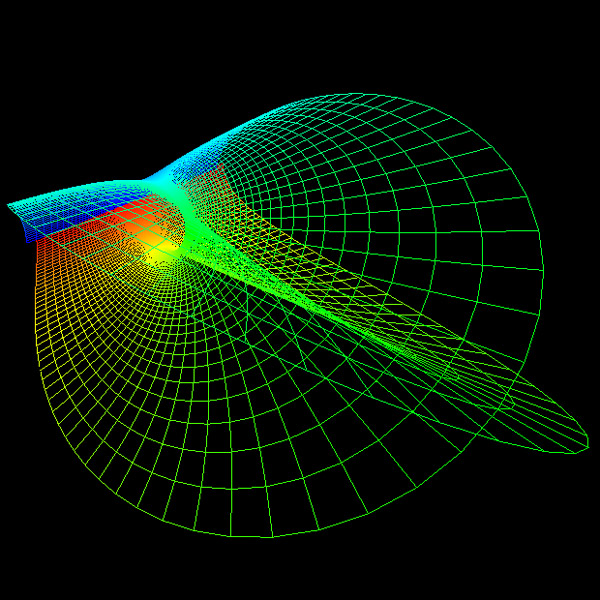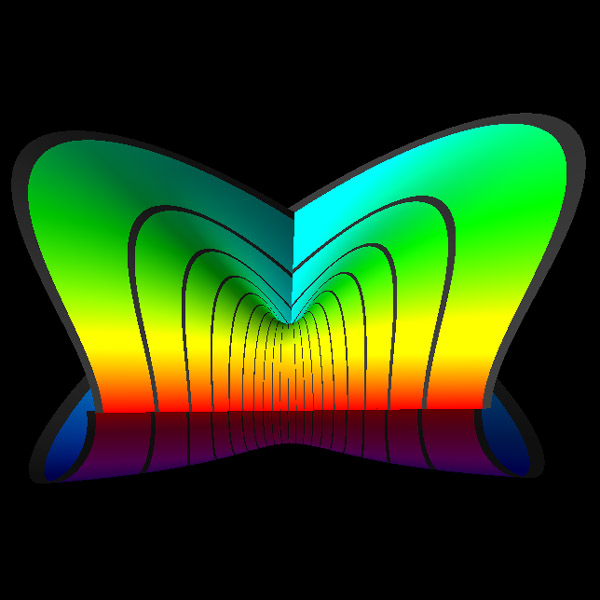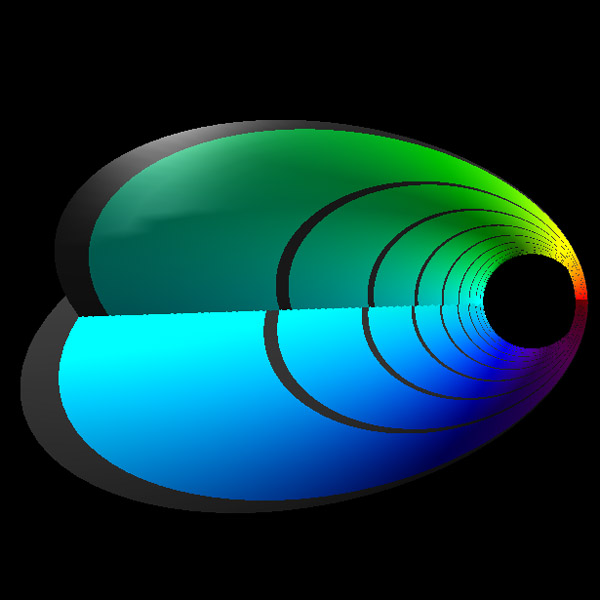# Cross Hole

By Roger Bagula

Graphics by Paul Bourke
January 2004

x = cos(u) / (R tan(v))
y = cos(2 u) / R
z = sin(2 u) / R
Where
R = cos2(u) cos2(v) + sin2(v)
and
0 <= u <= pi, 0 < v < pi